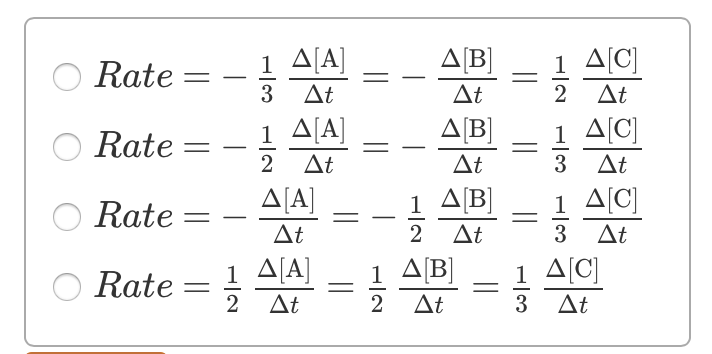# Problem: Consider the reaction: 2A(g)+B(g) → 3C(g).Part A) Determine the expression for the rate of the reaction with respect to each of the reactants and products.Part B) When A is changing at a rate of -0.160 M-s-1 , how fast is B changing?Part C) How fast is C increasing?

###### FREE Expert Solution

Recall that for a reaction aA  bB, the rate of a reaction is given by:

$\overline{){\mathbf{Rate}}{\mathbf{=}}{\mathbf{-}}\frac{\mathbf{1}}{\mathbf{a}}\frac{\mathbf{\Delta }\mathbf{\left[}\mathbf{A}\mathbf{\right]}}{\mathbf{\Delta t}}{\mathbf{=}}\frac{\mathbf{1}}{\mathbf{b}}\frac{\mathbf{\Delta }\mathbf{\left[}\mathbf{B}\mathbf{\right]}}{\mathbf{\Delta t}}}$

where:

Δ[A] = change in concentration of reactants or products (in mol/L or M), [A]final – [A]initial

Δt = change in time, tfinal – tinitial

We are given the following reaction

2A(g) + B(g) → 3C(g).

95% (125 ratings)###### Problem Details

Consider the reaction: 2A(g)+B(g) → 3C(g).

Part A) Determine the expression for the rate of the reaction with respect to each of the reactants and products.Part B) When A is changing at a rate of -0.160 M-s-1 , how fast is B changing?

Part C) How fast is C increasing?

Frequently Asked Questions

What scientific concept do you need to know in order to solve this problem?

Our tutors have indicated that to solve this problem you will need to apply the Average Rate of Reaction concept. You can view video lessons to learn Average Rate of Reaction. Or if you need more Average Rate of Reaction practice, you can also practice Average Rate of Reaction practice problems.